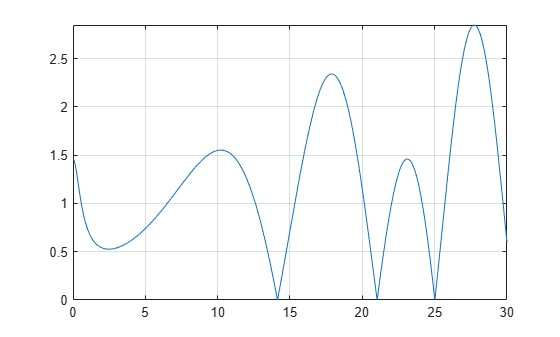zeta

Riemann zeta function

Description

example

zeta(z) evaluates the Riemann zeta function at the elements of z, where z is a numeric or symbolic input.

example

zeta(n,z) returns the nth derivative of zeta(z).

Examples

Find Riemann Zeta Function for Numeric and Symbolic Inputs

Find the Riemann zeta function for numeric inputs.

zeta([0.7 i 4 11/3])
ans =
-2.7784 + 0.0000i   0.0033 - 0.4182i   1.0823 + 0.0000i   1.1094 + 0.0000i

Find the Riemann zeta function symbolically by converting the inputs to symbolic objects using sym. The zeta function returns exact results.

zeta(sym([0.7 i 4 11/3]))
ans =
[ zeta(7/10), zeta(1i), pi^4/90, zeta(11/3)]

zeta returns unevaluated function calls for symbolic inputs that do not have results implemented. The implemented results are listed in Algorithms.

Find the Riemann zeta function for a matrix of symbolic expressions.

syms x y
Z = zeta([x sin(x); 8*x/11 x + y])
Z =
[        zeta(x), zeta(sin(x))]
[ zeta((8*x)/11),  zeta(x + y)]

Find Riemann Zeta Function for Large Inputs

For values of |z|>1000, zeta(z) might return an unevaluated function call. Use expand to force zeta to evaluate the function call.

zeta(sym(1002))
expand(zeta(sym(1002)))
ans =
zeta(1002)
ans =
(1087503...312*pi^1002)/15156647...375

Differentiate Riemann Zeta Function

Find the third derivative of the Riemann zeta function at point x.

syms x
expr = zeta(3,x)
expr =
zeta(3, x)

Find the third derivative at x = 4 by substituting 4 for x using subs.

expr = subs(expr,x,4)
expr =
zeta(3, 4)

Evaluate expr using vpa.

expr = vpa(expr)
expr =
-0.07264084989132137196244616781177

Plot Zeros of Riemann Zeta Function

Zeros of the Riemann Zeta function zeta(x+i*y) are found along the line x = 1/2. Plot the absolute value of the function along this line for 0<y<30 to view the first three zeros.

syms y
fplot(abs(zeta(1/2+1i*y)),[0 30])
grid onInput Arguments

collapse all

Input, specified as a number, vector, matrix or multidimensional array, or a symbolic number, variable, vector, matrix, multidimensional array, function or expression.

Order of derivative, specified as a nonnegative integer.

collapse all

Riemann Zeta Function

The Riemann zeta function is defined by

$\zeta \left(z\right)=\sum _{k=1}^{\infty }\frac{1}{{k}^{z}}$

The series converges only if the real part of z is greater than 1. The definition of the function is extended to the entire complex plane, except for a simple pole z = 1, by analytic continuation.

Tips

• Floating point evaluation is slow for large values of n.

Algorithms

The following exact values are implemented.

• $\zeta \left(0\right)=-\frac{1}{2}$

• $\zeta \left(1,0\right)=-\frac{\mathrm{log}\left(\pi \right)}{2}-\frac{\mathrm{log}\left(2\right)}{2}$

• $\zeta \left(\infty \right)=1$

• If $z<0$ and z is an even integer, $\zeta \left(z\right)=0.$

• If $z<0$ and z is an odd integer

$\zeta \left(z\right)=-\frac{\mathrm{bernoulli}\left(1-z\right)}{1-z}$

For $z<-1000$, zeta(z) returns an unevaluated function call. To force evaluation, use expand(zeta(z)).

• If $z>0$ and z is an even integer

$\zeta \left(z\right)=\frac{{\left(2\pi \right)}^{z}|\mathrm{bernoulli}\left(z\right)|}{2z!}$

For $z>1000$, zeta(z) returns an unevaluated function call. To force evaluation, use expand(zeta(z)).

• If $n>0$, $\zeta \left(n,\infty \right)=0.$

• If the argument does not evaluate to a listed special value, zeta returns the symbolic function call.